# Apply Trigonometry Knowledge to 3D Shapes

In this worksheet, students will be able to apply their knowledge of trigonometry to 3D shapes.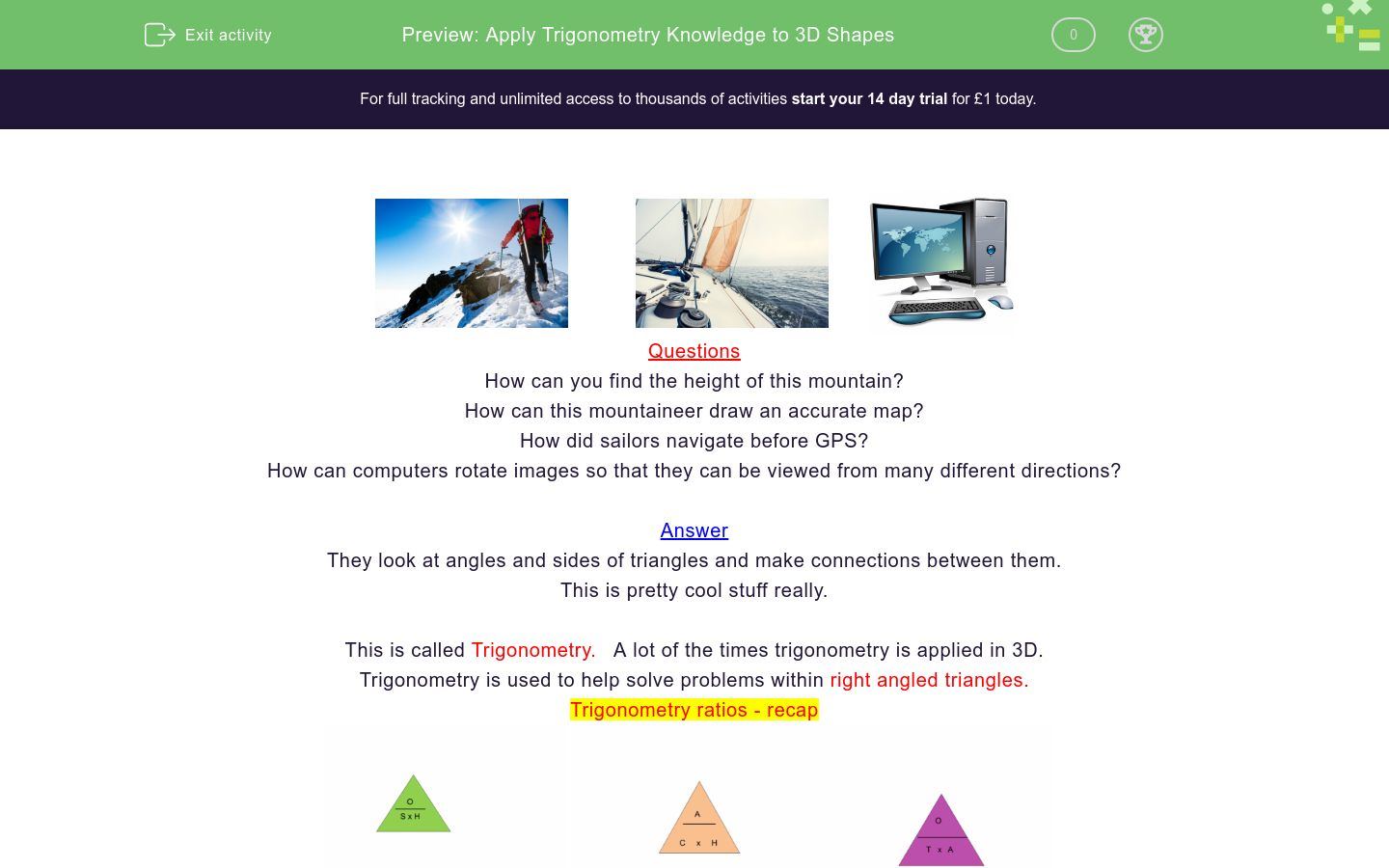Key stage:  KS 4

GCSE Subjects:   Maths

GCSE Boards:   AQA, Eduqas, OCR, Pearson Edexcel

Curriculum topic:   Geometry and Measures, Mensuration

Curriculum subtopic:   Mensuration and Calculation, Triangle Mensuration

Difficulty level:### QUESTION 1 of 10Questions

How can you find the height of this mountain?

How can this mountaineer draw an accurate map?

How did sailors navigate before GPS?

How can computers rotate images so that they can be viewed from many different directions?

They look at angles and sides of triangles and make connections between them.

This is pretty cool stuff really.

This is called Trigonometry.   A lot of the times trigonometry is applied in 3D.

Trigonometry is used to help solve problems within right angled triangles.

Trigonometry ratios - recap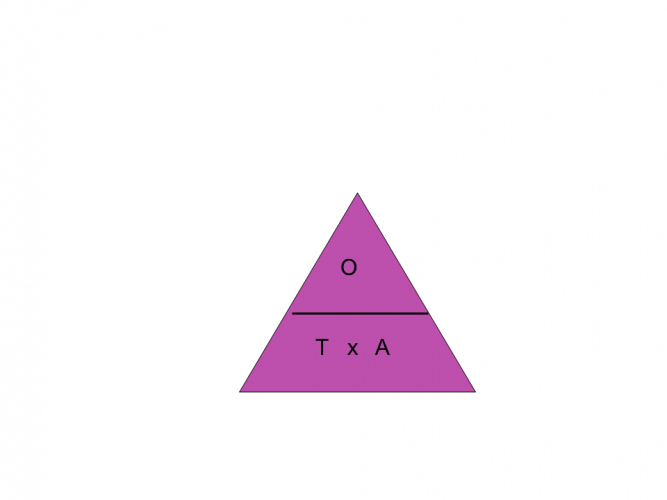Example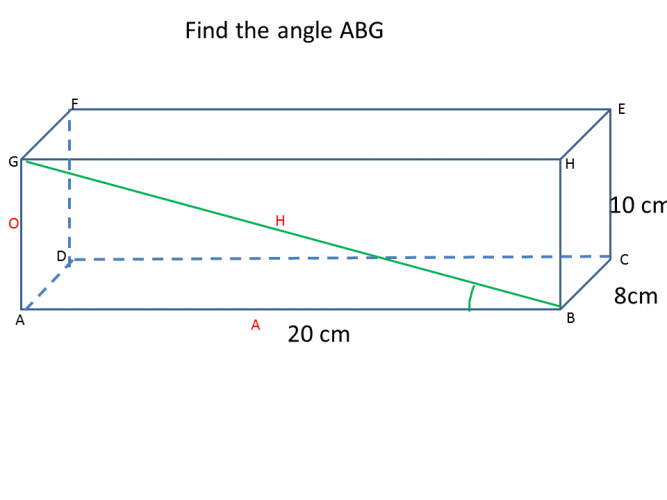1. Draw in the right angled triangle as shown in the example.  Then if it is helpful draw it out in 2 dimensions.

2. Label the triangles

3. Apply the correct trig ratio

4.  We have the opposite 10 cm and the adjacent 20 cm, therefore the tan ratio.

5.  10 ÷ 20 = 0.5 then tan-1 0.5 = 26.6° correct to one decimal place.

So time to quit the computer game (although trigonometry is used to help create them) and have a go at applying your knowledge.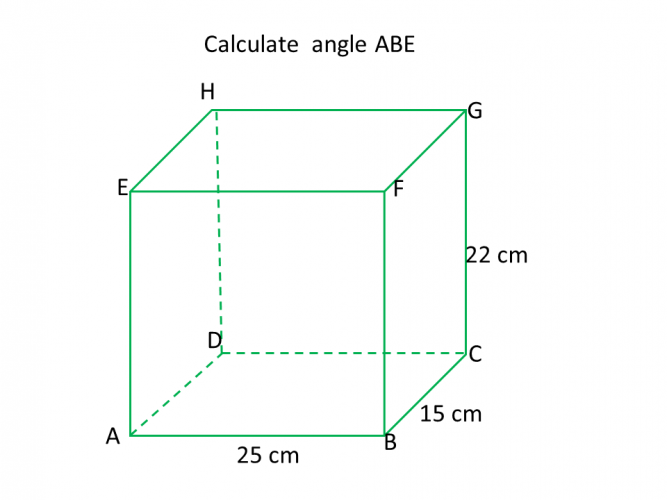88°

48.6°

41.3°

28.35°88°

48.6°

41.3°

28.35°47.7° 45.5° 42.2° Angle ACB is26.5°

24.3°

22.6°

25.4°The length of DB is 21.6°, 20.9° or 18.4°.29.6 cm 30.2 cm 22.9 cm 25.9 cm The length of AH is29.6 cm 30.2 cm 22.9 cm 25.9 cm The length of AH is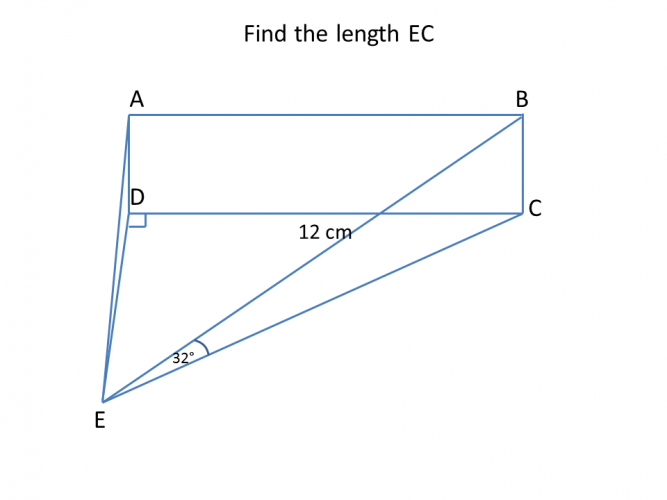• Question 141.3°
EDDIE SAYS
Could you spot the angle okay? Don't forget to draw the line in to find it. Visualising the shape makes things easier. 22 ÷ 25 = 0.88 tan-1 0.88 = 41.3° correct to 1 decimal place.
• Question 2EDDIE SAYS
Remember the angle is the middle letter of the three that you are looking for. If in doubt sketch it out. There is an awful lot to take in to try and do this in your head. 15 ÷ 18 = 0.8333... tan-1 0.8333 = 39.8°
• Question 321.8
EDDIE SAYS
Why was this angle freezing? It was less than 32°. I hope that is what you got anyway. 12 ÷ 30 = 0.4 tan-1 0.4 = 21.8° correct to 1 decimal place.
• Question 447.7° 45.5° 42.2° Angle ACB is
EDDIE SAYS
And just when you got used to cubes and cuboids, the pyramid pops up. 20 ÷ 22 = 0.909.... tan-1 0.909 = 42.2° Correct to 1 decimal place. The dimensions for the pyramid stage at Glastonbury will be calculated using trigonometry, so you see it gets everywhere.
• Question 526.5°
EDDIE SAYS
Are you getting used to seeing the 2d shape within the 3d shape? I hope so. By finding them you can break down a question so much easier. 10 ÷ 20 = 0.5 tan-1 0.5 = 26.5° correct to 1 decimal place.
• Question 657.3
EDDIE SAYS
Why are acute angles always a bit sad? Because they are never right..... I hope you are happy with your progress in this area. It is just a case of practice, practice and practice. 14 ÷ 9 = 1.55555 tan-1 1.5555 =57.3° correct to 1 decimal place.
• Question 7The length of DB is 21.6°, 20.9° or 18.4°.
EDDIE SAYS
12 ÷ sin35 = 20.9 cm correct to 1 decimal place. When finding a side you may multiply or divide unlike when finding an angle you always divide.
• Question 829.6 cm 30.2 cm 22.9 cm 25.9 cm The length of AH is
EDDIE SAYS
Oh this is a bit different a length instead of an angle. No worries to you as long as you know your trig ratios and have labelled the triangle correctly. I find it easier to draw out the 2d shape first and then put the angle in. 18 ÷ cos 46 = 25.9 cm correct to 1 decimal place. This makes sense as you are looking for the hypotenuse which is the longest side.
• Question 9EDDIE SAYS
Hopefully this becoming second nature to you now. Cubes, cuboids, pyramids, prisms..no problem. 9 ÷ sin28 = 19.2 correct to 1 decimal place.
• Question 1010.2
EDDIE SAYS
I hope you are feeling a little more confident now with trigonometry in 3d. All you need to do is study the diagrams carefully to pick out the correct sides or angles and you are halfway there. cos 32 x 12 = 10.2 cm correct to 1 decimal place.
---- OR ----

Sign up for a £1 trial so you can track and measure your child's progress on this activity.

### What is EdPlace?

We're your National Curriculum aligned online education content provider helping each child succeed in English, maths and science from year 1 to GCSE. With an EdPlace account you’ll be able to track and measure progress, helping each child achieve their best. We build confidence and attainment by personalising each child’s learning at a level that suits them.

Get started